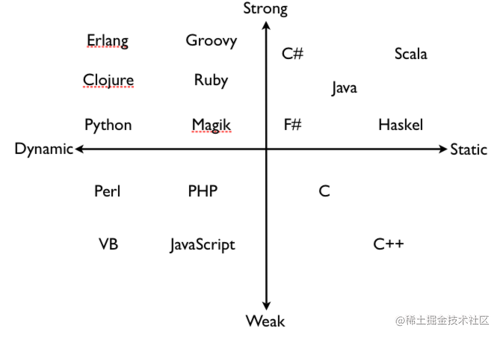# JavaScript 是動態弱型別語言，TypeScript 呢？

## 型別系統### 強型別

• 語言層面限制函式的實參型別必須與形參型別相同
• 強型別語言中不允許任意的隱式型別轉換

• 錯誤更早暴露
• 程式碼提示更智慧
• 編碼更準確重構更牢靠
• 減少不必要的型別判斷

### 弱型別

• 弱型別語言層面不會限制實參的型別
• 弱型別語言允許任意的資料隱式型別轉換

### 總結

```tsx let num1: number = 1; num1 = '1'; // error TS2322: Type 'string' is not assignable to type 'number'.

let str: string = 'str'; let num2: number = 2; str = str + num2; // str2 ```

```jsx let cc = {}; cc = '1';

let aa = '123'; let bb = true; aa = aa * bb; // 123 ```

```tsx let aa: string = '123'; let bb: number = 1; console.log(aa * bb); // The left-hand side of an arithmetic operation must be of type 'any', 'number', 'bigint' or an enum type.```

（參見 Oracle 文件：第五章：型別轉換

```java int x=20; boolean y=true; System.out.println("printing: " + x + y); // printing: 20true

// Java 編譯器對程式碼進行重寫 int x=20; boolean y=true; System.out.println("printing: " + new Integer(x).toString() + Boolean.toString(y)); ```